Astro indicators in Timing Solution

� 2007 Sergey Tarassov

Introduction

Technology description - synthesis

How to create an indicator to reveal turning points

Introduction

With Timing Solution, it is possible to calculate any of the most popular astro indicators. Now (September 2007) there are 17 variants of different astro indicators. You will find among them the indicators well known and described in different open sources (such as Barco, Ganue, and Hoff indicators and their variations) as well as the indicators created by me according to suggestions of the software users. In this article I will do a brief overview of these indicators and show how to customize them for any financial instrument. My goal was to analyze how these indicators forecast the turning points and volatility. The Dow Jones Industrial index data from 1885 to August 2007 were used. To analyze volatility, I used the data from the year 1980. The orb for anything planetary-related in this research is 2 degrees.

1. Planetary Pictures Geo (0..180) scale. To calculate this index, the program takes all angles between the planets in geocentric using 0..180 degrees scale. This index shows how many planetary pairs have the same angle separation. I did not find any correlation and price for this index. It does not work for DJI.

2. Planetary Pictures Helio (0..180) scale.. It is calculated the same way as the previous indicator, the only difference is the use of heliocentric planetary positions. It shows some impact on volatility, especially when the value of this indicator is small (less than 10).

3. Planetary Pictures Geo (0..360) scale. It calculates the angle between planets using 0...360 degrees scale. It shows how many planetary pairs have the same angle separation (0..360 degrees scale). No effect found.

4. Planetary Pictures Helio (0..360) scale. It works similar to #2 indicator

5. Sum of angle separation (-180...+180) Geo. It calculates the sum for all angles between the planets. The program assigns a positive value to the front position, while the back position has a negative value. No effect found.

6. Sum of angle separation (-180...+180) Helio. This indicator definitely correlates to the top turning points. When the value of this indicator falls into -400 to +400 range, the probability of a top turning point is high.

7. Sum of absolute separation 0..180 degrees scale Geo. It calculates the sum of the angles between planets using 0..180 scale, back and front positions have the same value. The value in the range -500..-1000 is related to a bottom turning point.

8. Sum of absolute separation 0..180 degrees scale Helio. The value in the range -150..+300 is related to the top turning point.

9. Sum of absolute separation 0..360 degrees scale Geo. No effect found.

10. Sum of absolute separation 0..360 degrees scale Helio. No effect found.

11. Amount of midpoint conjunctions Geo. To calculate this indicator, the program takes all midpoints between the planets and counts the amount of midpoints that have the same longitude. The value between +55 and +120 is related to the top turning point.

12. Amount of midpoint conjunctions Helio. No effect found.

13. Amount of midpoint oppositions Geo. The value between 0 .. +10 is related to the bottom turning point.

14. Amount of midpoint oppositions Helio. No effect found.

15. Index of cyclical variations Geo. It calculates the amount of waxing angles minus the amount of waning angles. It is a strong indicator. The value between +20 and +35 is related to the top turning points, while the value -9..-21 is related to the bottom.
Also sometimes this index correlates with the trend: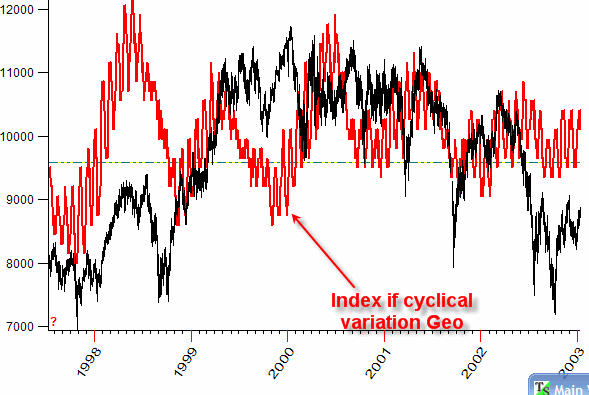16. Index of cyclical variations Helio. It may forecast bottom turning point when its value is in the range -8..-16.

17. Hoff index. The negative value of this index correlates very well with the trend.

I have added several new models to the program based on this research. Timing Solution users will find these new ULE models in the upgrade of September 12, 2007. Here is the list:

1) A model to forecast top turning points.

2) A model to forecast bottom turning points.

3) Trend index based on "Index of cyclical variations Geo"

4) Trend index based on Hoff index.

You can run these models here: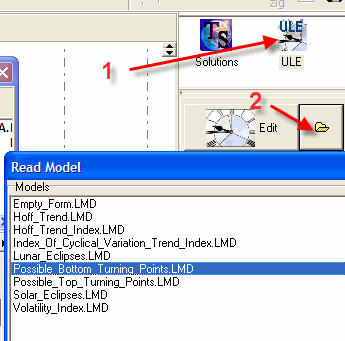Remember that you can use these indicators as hints only.

The most common mistake

Before any discussion, I would like to talk about a very common mistake that takes place when somebody applies/invents any astro indicator.

Let us consider planetary pictures heliocentric indicators (What follows below, can be applied to any indicator). This indicator simply shows the amount of planetary pairs in heliocentric system that have the same angle separation.

There is no mistake yet. It comes later.

If my goal would be to sell you this indicator, I would start an advertising campaign with something like this: "Look at the price chart and the indicator displayed together: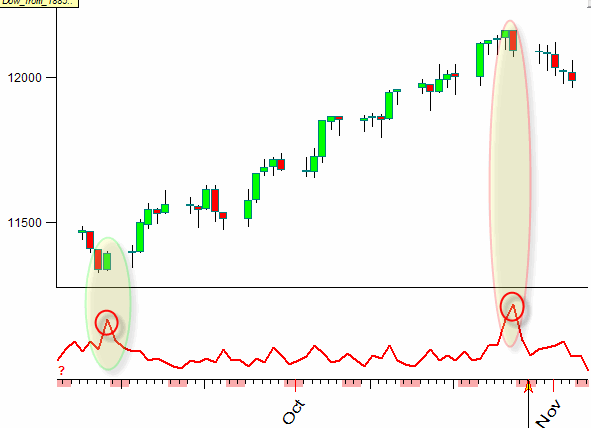You see that the big values of this indicator (see the red curve at the bottom) correspond to the major turning points on the price chart ".

There is still no mistake. The calculation is OK for the chosen time interval, and the conclusion is OK. You may be impressed and buy this indicator. And - it is not OK when you apply it for your trading.

Does it sounds familiar? I have seen it so many times in the letters from my users. What is wrong? Where is the mistake?

It is there. I would call it misrepresentation (maybe, unwilling or unintended).

If the picture above is the only piece of information regarding this indicator, we are not actually able to figure out how this indicator will perform. See yourself.

Let us take next two consequent intervals that follow the picture shown above. Here are the price chart and that same indicator together, though without any selection: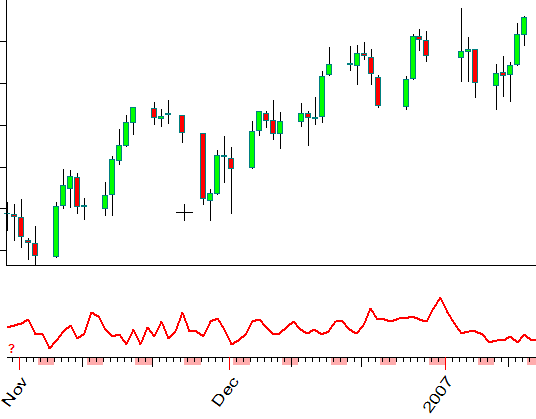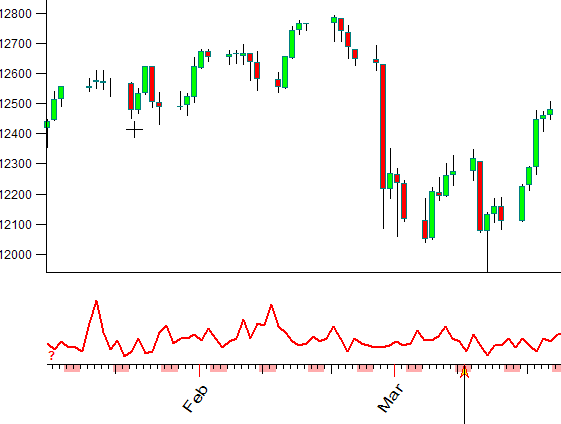Could you please make the same conclusion regarding the indicator and the price turning point NOW? I do not see any connection between the price  and the analyzed indicator.

I could easily do the same thing for volatility or any other index - taking just one good example and making a conclusion that "the high value of our astro indicator tends to increase/decrease volatility (or whatever we research)". Choosing the appropriate time span, we can approve/disapprove anything.

To understand  how any astro indicator (or any indicator) works, it is necessary to analyze many subsequent intervals without any selection.

The detailed statistical analysis shows that often these indicators work totally different from what we are expecting from them. For example if we modify the above mentioned indicator using the geocentric system (not heliocentric), we will find that its high value tends to appear together with TOP turning points, not bottoms. Thus the high value of this indicator can serve as a sign (with some probability, of course) for the upcoming TOP turning point.

In this article I will show you how to analyze and create the astro indicator using Timing Solution software.

Technology description - analysis

In Timing Solution you can create practically any known astro indicator. Look at "Astro Indicators" tab. You will find there 17 the most used indicators including Ganue, Barbo, Hoff indicators and the indicators based on Planetary Pictures, calculated for Geo and Helio systems.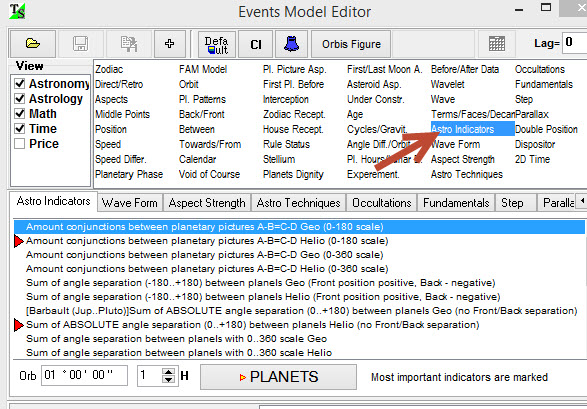You may display any or several indicators together with the price chart: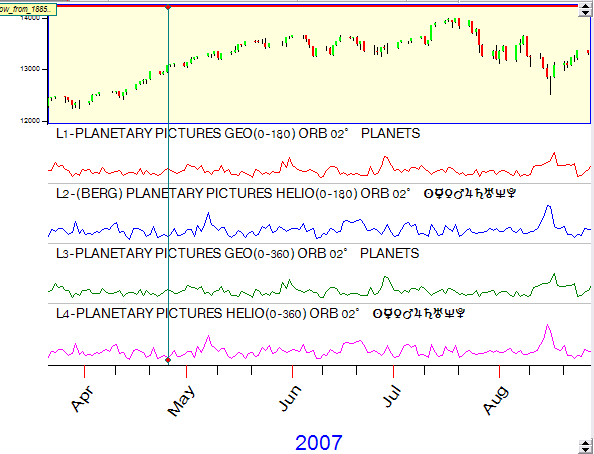Let us perform the statistical analysis for any of these indicators. Our goal is to get the objective picture of how this indicator actually works. Let us analyze "Index of cyclical variation":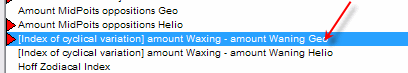This indicator shows a balance of waxing and waning angles between the planets in geocentric system. Click this button: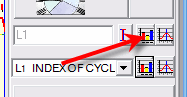to open the statistical module. You will get the scattered diagram: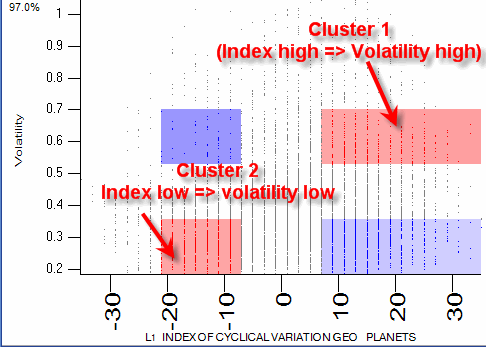On X axis the program shows the value of "Index of cyclical variation" for all price bars, while Y axis shows the appropriate value of Volatility index. Actually you can analyze any index here (I tried RSI, ADX and other):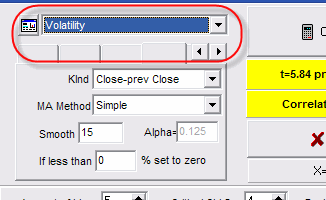I have analyzed Dow Jones Industrial index using the data from the year 1980 to 2007. The program draws more than 7000 points on this diagram. For us the most interesting thing is the existence of the red regions. You can see on the diagram two red clusters that provide us a valuable information regarding the effect that this index has on volatility.

Cluster N1 shows what happens when this index is high. If our astro indicator is higher than 7 (which means that there are more waxing angles between the planets than waning ones), the volatility is high enough: 0.53-0.7%. We can accept this fact as a statistically verified one - the big amount of waxing angles between the planets leads to the high volatility of the Dow Jones Index.

You can see there the second cluster. It corresponds to the negative value of this index, and it shows the small values of volatility, no more than 0.34%. In other words, when there are more waning angles between the planets, the volatility is low.

You can play with the statistical parameters (especially with Chi Square and Min amount of points in the bin):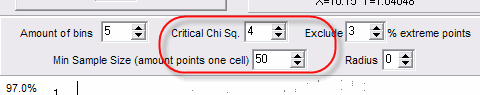It allows you to understand what clusters are the strongest ones.

Technology description - synthesis

Now we have a tool that allows us easily find out how any astro indicator affects the stock market. Look at the table below. There I put the results of my analysis of 10 different indicators. These 10 indicators are the most influential for volatility. The values there are the values of the indicator that correspond to high/low volatility:

 Indicator Volatility High Volatility Low L1=Sum of angle separation (-180..+180) Geo +800..+4000 -600..-4000 L2=Sum of angle separation (-180..+180) Helio +600 ... +1500 -300 ... -1300 L3=Sum of ABSOLUTE angle separation (0..+180) Helio +600 ... +1000 -1000 ... -2000 L4=Sum of angle separation  0...360 Geo +800 ... +2300 -800 ... -2300 L5=Amount midpoints conjunctions Helio 0 ... +30 +30 ... +60 L6=Amount midpoints opposition Geo +20 ... +30 L7=Amount midpoints opposition Helio +10 ... +20 L8=Index of Cyclical variation Geo +7 ... +40 -7 .. -21 L9=Index of Cyclical variation Helio +4 ... +15 L10=Hoff Zodiacal Index +2 .. +42

How did I get this information? For example, the first line of this table is received from this diagram: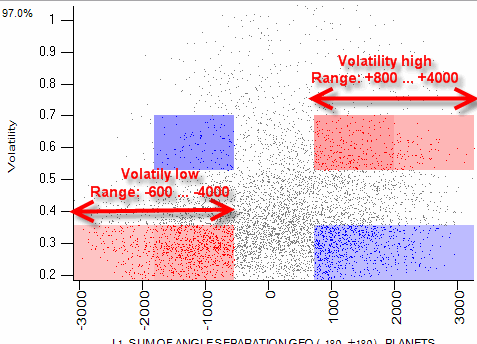These values for all 10 indicators are statistically verified. Therefore, I can take each one of them as the meaningful event and add them to the ULE module of the program (ULE stands for Universal Language of Events, I include there things that play some role for the stock market):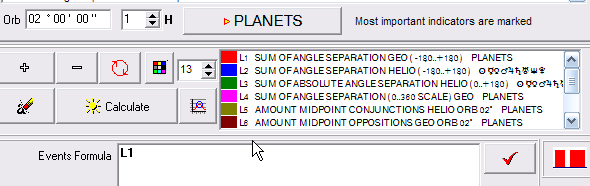Now let us create the final formula that summarizes our newly found knowledge about these indexes (indicators).

Consider the first line:

 L1=Sum of angle separation (-180..+180) Geo +800..+4000 -600..-4000

The message that we get here is simple: DJI is volatile when the first indicator is in the range between (800, 4000) and (-600, -4000). Volatility grows when the indicator is closer to (800, 4000); it decreases when the indicator is negative (-600, -4000).

Let us write this condition together: L1 - this is the analyzed indicator. To write "Volatility is high when the indicator between 800 and 4000", use this expression: Range(L1,800,4000).

Next we need to write this:  "Volatility is low when the indicator is between -600 and -4000". Do the subtraction: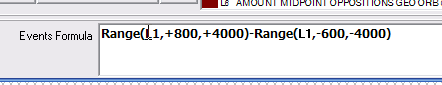Then perform the same procedure for the next indicator:

 L2=Sum of angle separation (-180..+180) Helio +600 ... +1500 -300 ... -1300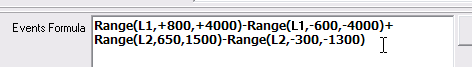Add it to the first one.

Finally you will get the formula that involves all analyzed terms. See how this indicator looks (red - our indicator, blue - actual volatility):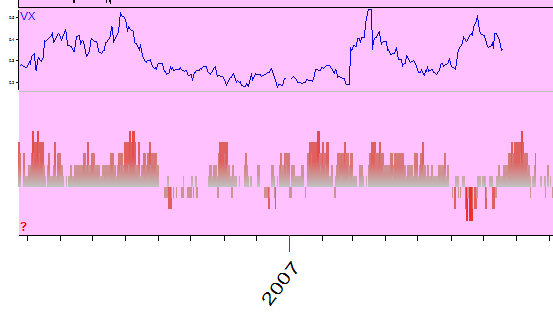Of course it is not an ideal, I would not recommend it to you as your major trading tool. However, its quality is a lot better than that of any indicator by itself.

While you are working with this complicated formula, I recommend to set this option OFF to hide all terms that our indicator consists of: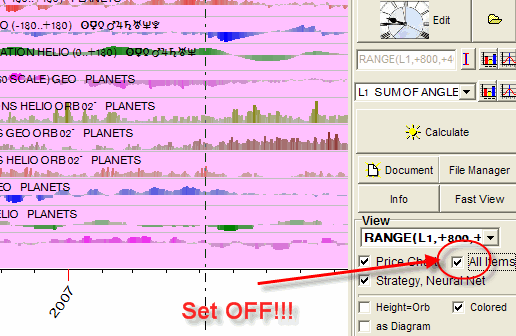How to create an indicator to reveal turning points

The technology is exactly the same as above. The only difference is that instead of Volatility index in the scattered diagram use a detrended zigzag index: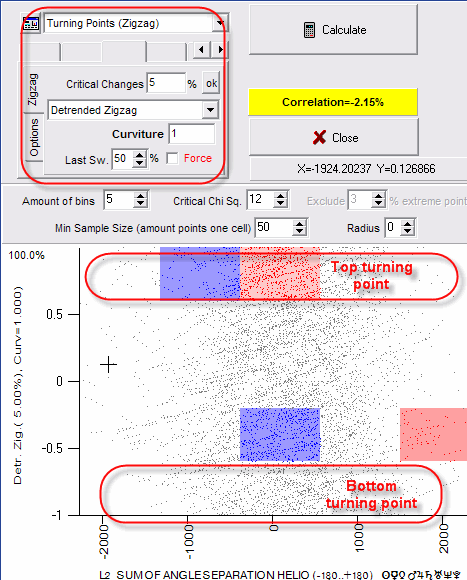Here the top part of diagram corresponds to the top turning points, the bottom - to the bottom turning points. Thus the upper red cluster can be interpreted as this: when the analyzed index is in the range -300 to +500, the up turning point is more probable.

� 2007 Timing Solution

September 12, 2007